• Java 八种基本类型包装类
千次阅读
2021-03-09 15:37:10

基本数据类型 　　包装类型

----------------------------------------------

byte　　　  java.lang.Byte(父类Number)

short　　　 java.lang.Short(父类Number)

int 　　　　 java.lang.Integer(父类Number)

long 　　　 java.lang.Long(父类Number)

float 　　　 java.lang.Float(父类Number)

double 　　 java.lang.Double(父类Number)

boolean　　java.lang.Boolean(父类Object)

char 　　　 java.lang.Character(父类Object)

Number类中有这样的方法：

byte byteValue() 以 byte 形式返回指定的数值。

abstract double doubleValue()以 double 形式返回指定的数值。

abstract float floatValue()以 float 形式返回指定的数值。

abstract int intValue()以 int 形式返回指定的数值。

abstract long longValue()以 long 形式返回指定的数值。

short shortValue()以 short 形式返回指定的数值。

这些方法其实所有的数字包装类的子类都有，这些方法是负责拆箱的。

// 基本数据类型 -(转换为)->引用数据类型(装箱)

Integer i = new Integer(123);

// 将引用数据类型--(转换为)-> 基本数据类型

float f = i.floatValue();

System.out.println(f); //123.0

// 将引用数据类型--(转换为)-> 基本数据类型(拆箱)

int retValue = i.intValue();

System.out.println(retValue); //123

java5 之后 自动装箱和拆箱

[-128到127]之间的数据比较特殊

public class IntegerTest01 {

public static void main(String[] args) {

Integer a = 128;

Integer b = 128;

System.out.println(a == b); //false

/*

java中为了提高程序的执行效率，将[-128到127]之间所有的包装对象提前创建好，

放到了一个方法区的“整数型常量池”当中了，目的是只要用这个区间的数据不需要

再new了，直接从整数型常量池当中取出来。

原理：x变量中保存的对象的内存地址和y变量中保存的对象的内存地址是一样的。

*/

Integer x = 127;

Integer y = 127;

// == 永远判断的都是两个对象的内存地址是否相同。

System.out.println(x == y); //true

}

}

总结一下之前所学的经典异常？

空指针异常：NullPointerException

类型转换异常：ClassCastException

数组下标越界异常：ArrayIndexOutOfBoundsException

数字格式化异常：NumberFormatException

//网页上文本框中输入的100实际上是"100"字符串。后台数据库中要求存储100数字，此时java程序需要将"100"转换成100数字。

int retValue = Integer.parseInt("123"); // String -转换-> int

//int retValue = Integer.parseInt("中文"); // NumberFormatException

System.out.println(retValue + 100);

// valueOf方法作为了解

//static Integer valueOf(int i)

// 静态的：int-->Integer

Integer i1 = Integer.valueOf(100);

System.out.println(i1);

// static Integer valueOf(String s)

// 静态的：String-->Integer

Integer i2 = Integer.valueOf("100");

System.out.println(i2);

三种类型的转换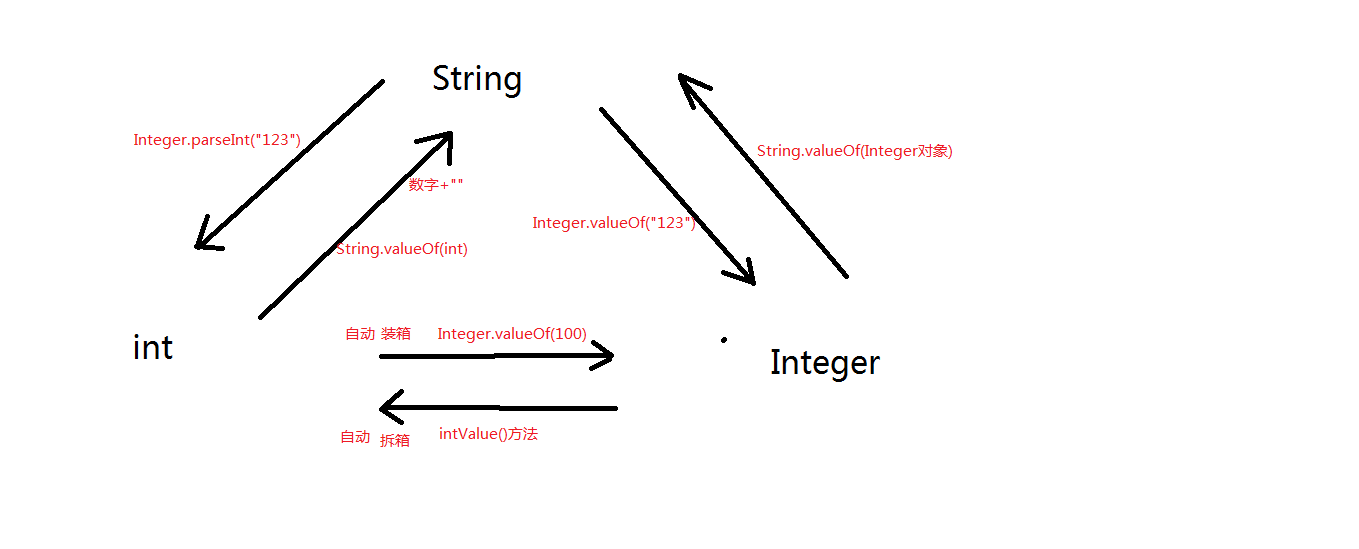更多相关内容
• 一、8基本数据类型对应的包装类型名 基本数据类型 包装类型 byte java.lang.Byte（父类Number） short java.lang.Short（父类Number） int java.lang.Integer（父类Number） long java.lang.Long...

# 一、8种基本数据类型对应的包装类型名

基本数据类型包装类型
bytejava.lang.Byte（父类Number）
shortjava.lang.Short（父类Number）
intjava.lang.Integer（父类Number）
longjava.lang.Long（父类Number）
floatjava.lang.Float（父类Number）
doublejava.lang.Double（父类Number）
booleanjava.lang.Boolean（父类Object）
charjava.lang.Character（父类Object）

注意： 8种包装类属于 引用数据类型

# 二、父类Number方法

方法名作用
byte byteValue()byte 形式返回指定的数值
abstract double doubleValue()double 形式返回指定的数值
abstract float floatValue()float 形式返回指定的数值
abstract int intValue()int形式返回指定的数值
abstract long longValue()long形式返回指定的数值
short shortValue()short形式返回指定的数值

注意： 这些方法所有的数字包装类的子类都有，这些方法是负责 拆箱 的。

# 三、手动装箱/手动拆箱

public class IntegerTest02 {
public static void main(String[] args) {

// 123这个基本数据类型，进行构造方法的包装达到了：基本数据类型向引用数据类型的转换。
// 基本数据类型 -(转换为)->引用数据类型（装箱）
Integer i = new Integer(123);

// 将引用数据类型--(转换为)-> 基本数据类型
float f = i.floatValue();
System.out.println(f); //123.0

// 将引用数据类型--(转换为)-> 基本数据类型（拆箱）
int retValue = i.intValue();
System.out.println(retValue); //123
}
}


# 四、构造方法（Java9后过时）

Integer构造方法名
Integer(int value)
Integer(String s)

注意：

• Byte、Short、Long、Float、Double、Boolean的构造方法和Integer的构造方法一样。
• Character只有一个构造方法
Character构造方法名
Character(char value)

注意： Float比Integer多一个

Float构造方法名
Float(double value)

eg.

public class IntegerTest03 {
public static void main(String[] args) {

// Java9之后不建议使用这个构造方法了。出现横线表示已过时。
// 将数字100转换成Integer包装类型（int --> Integer）
Integer x = new Integer(100);
System.out.println(x);

// 将String类型的数字，转换成Integer包装类型。（String --> Integer）
Integer y = new Integer("123");
System.out.println(y);

// double -->Double
Double d = new Double(1.23);
System.out.println(d);

// String --> Double
Double e = new Double("3.14");
System.out.println(e);
}
}


# 五、包装类常量

常量属性
MAX_VALUE
MIN_VALUE

注意： Byte、Short、Integer、Long、Float、Double都有。

eg.

public class IntegerTest04 {
public static void main(String[] args) {
// 通过访问包装类的常量，来获取最大值和最小值
System.out.println("int的最大值：" + Integer.MAX_VALUE);
System.out.println("int的最小值：" + Integer.MIN_VALUE);
System.out.println("byte的最大值：" + Byte.MAX_VALUE);
System.out.println("byte的最小值：" + Byte.MIN_VALUE);
}
}


# 六、自动装箱/自动拆箱（java5新特性）

## 1.什么是自动装箱和自动拆箱？

• 自动装箱：基本数据类型自动转换成包装类
• 自动拆箱：包装类自动转换成基本数据类型

## 2.自动装箱和自动拆箱的好处？

• 方便编程
• 有了自动拆箱之后，Number类中的方法就用不着了

eg.

public class IntegerTest05 {
public static void main(String[] args) {
// 900是基本数据类型
// x是包装类型
// 基本数据类型 --(自动转换)--> 包装类型：自动装箱
Integer x = 900; // 等同于：Integer z = new Integer(900);
System.out.println(x);

// x是包装类型
// y是基本数据类型
// 包装类型 --(自动转换)--> 基本数据类型：自动拆箱
int y = x;
System.out.println(y);
}
}


注意：自动装箱底层实际上还是new对象了。

public class IntegerTest05 {
public static void main(String[] args) {
// z是一个引用，z是一个变量，z还是保存了一个对象的内存地址。
Integer z = 1000; // 等同于：Integer z = new Integer(1000);

// 分析为什么这个没有报错呢？
// +两边要求是基本数据类型的数字，z是包装类，不属于基本数据类型，这里会进行自动拆箱。将z转换成基本数据类型
// 在java5之前你这样写肯定编译器报错。
System.out.println(z + 1);//1001

Integer a = 1000; // Integer a = new Integer(1000); a是个引用，保存内存地址指向对象。
Integer b = 1000; // Integer b = new Integer(1000); b是个引用，保存内存地址指向对象。
System.out.println(a == b); //false
}
}


注意：

• == 这个运算符不会触发自动拆箱机制
• == 永远判断的都是两个对象的内存地址是否相同。
• 只有 + - * / 等运算的时候才会触发自动拆箱机制

# 七、（方法区）整数型常量池

public class IntegerTest06 {
public static void main(String[] args) {
Integer a = 128;
Integer b = 128;
System.out.println(a == b); //false

//原理：x变量中保存的对象的内存地址和y变量中保存的对象的内存地址是一样的。
Integer x = 127;
Integer y = 127;
// == 永远判断的都是两个对象的内存地址是否相同。
System.out.println(x == y); //true
}
}


java中为了提高程序的执行效率，将 [-128 ~ 127] 之间所有的包装对象提前创建好， 放到了一个方法区的“整数型常量池”当中了。

目的是：只要用这个区间的数据不需要再new了，直接从整数型常量池当中取出来。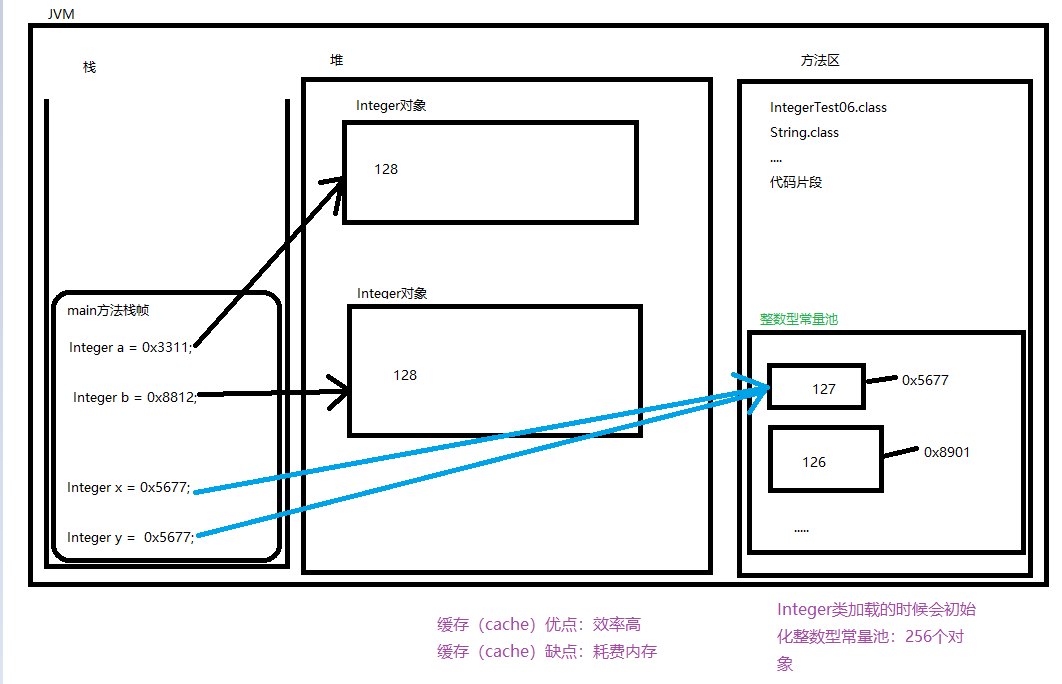# 八、Integer方法

方法名作用
static Integer decode(String nm)将String转成Integer
static int compare(int x, int y)比较两个数是否相等；相等返回0；前大后小返回1；后大前小返回-1
static int signum(int i)符号函数；负数返回-1；正数返回1；0返回0
static String toBinaryString(int i)将i转成二进制
static String toHexString(int i)将i转成十六进制
static String toOctalString(int i )将i转成八进制
常用方法
static int parseInt(String s)字符串转int
static Integer valueOf(String s)字符串转Integer
String toString()Integer转String
boolean equals(Object obj)判断两个Integer是否相等

注意： Byte、Short、Long、Float、Double、Boolean照葫芦画瓢，方法差不多相同。

eg.

class IntegerTest{
public static void main(String[] args) {
Integer d = Integer.decode("123");
System.out.println(d);//自动拆箱 123

Integer a = 100;
Integer b = 100;

int res1 = Integer.compare(a, b);
System.out.println(res1);//0
res1 = Integer.compare(-a, b);
System.out.println(res1);//-1
res1 = Integer.compare(a, -b);
System.out.println(res1);//1

System.out.println(a.equals(b));//true

int i = Integer.parseInt("123");
System.out.println(i);//123

System.out.println(Integer.signum(-123));//-1
System.out.println(Integer.signum(123));//1
System.out.println(Integer.signum(0));//0

System.out.println(Integer.toBinaryString(10));//1010
System.out.println(Integer.toOctalString(10));//12
System.out.println(Integer.toHexString(10));//a

String s = Integer.toString(123);
System.out.println(s);//123

Integer int1 = Integer.valueOf("123");
System.out.println(int1);//123
}
}


# 九、Character方法

方法名作用
char charValue()将Character转成char
int compareTo(Character anotherCharacter)判断两个Character是否相等；相等返回0；前大后小返回1；后大前小返回-1
常用方法
boolean equals(Object obj)判断两个Character是否相等
String toString()将Character转成String
static boolean isDigit(char ch)判断ch是不是数字
static boolean isLetter(char ch)判断ch是不是字母
static boolean isLetterOrDigit(char ch)判断ch是不是字母或数字
static boolean isLowerCase(char ch)判断ch是不是小写字母
static boolean isUpperCase(char ch)判断ch是不是大写字母
static boolean isSpaceChar(char ch)判断ch是不是空格
static Character valueOf(char c)将char转成Character

eg.

class CharacterTest{
public static void main(String[] args) {
Character c = 'a';

char res1 = c.charValue();
System.out.println(res1);//a

Character a = 'a';
Character b = 'b';
System.out.println(a.compareTo(b));//-1

System.out.println(a.equals(b));//false

System.out.println(Character.isDigit('1'));//true

System.out.println(Character.isLetter('a'));//true

System.out.println(Character.isLetterOrDigit('1'));//true
System.out.println(Character.isLetterOrDigit('a'));//true

System.out.println(Character.isLowerCase('a'));//true

System.out.println(Character.isUpperCase('A'));//true

System.out.println(Character.isSpaceChar(' '));//true

System.out.println(c.toString());//"a"

System.out.println(Character.valueOf('c'));//c

}
}

展开全文• java八种基本数据类型分为四类八种，四类分别为整型、浮点型、布尔型、字符型；八种分别为byte、short、int、long、float、double、boolean、char； java八种基本数据类型的字节数:分别为1、2、4、8个字节；1字节...

## 详解一、八种基本数据类型常识

### 1.1、基本常识表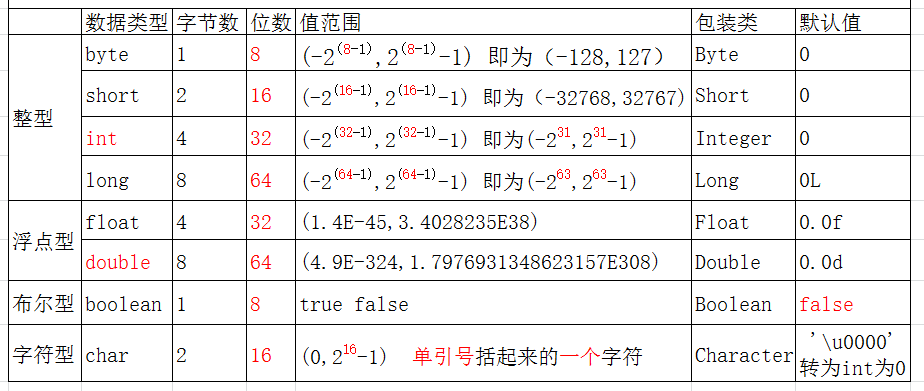对于上图有以下几点需要注意：

1. java八种基本数据类型分为四类八种，四类分别为整型、浮点型、布尔型、字符型；八种分别为byte、short、int、long、float、double、boolean、char；
2. java八种基本数据类型的字节数:分别为1、2、4、8个字节；1字节(byte、boolean)、 2字节(short、char)、4字节(int、float)、8字节(long、double)；
3. 整数的默认类型为int，浮点数的默认类型为double
4. 八种基本数据类型的包装类：除了char的是Character、int类型的是Integer，其他都是首字母大写
5. 关于值的范围问题，需要注意char类型是无符号的，不能为负，所以是0开始的；

## 详解二、直接量与类型转换

### 2.1、直接量

1. 整数型的直接量默认为int类型
2. 浮点型的直接量默认为double类型
@Test
public void d() {
int a=100;//这个100就是直接量
a=a+100;//但是这个a+100不是直接量
double b=3.14;
}

### 2.2、类型转换

1.自动转换：低类型的向高类型的转换

如下图：顺着箭头的方向是可以自动转换的；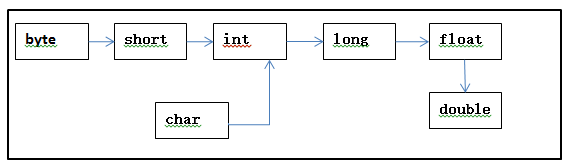2.强制转换：高类型的向底类型转换，但可能会数据溢出或者精度丢失

### 以上现象在实际中可以出现这三种问题：

• 定义变量时出现的类型转换
@Test
public void e(){
long a=200;//200是直接量，默认为int类型这里是自动转换为弄类型

/*long b=100000000000;*/
//100000000000是个整数直接量，默认应该为int类型，但是超出了int类型的取值范围

long c=1000000000000L;
//在整数直接量后面将了一个L，表示该直接量不再默认为int类型，为long类型，所以没错

/*float d=34.3;*/
//浮点型直接量默认为double,double类型大，所以不能直接转换为float

float e=34.3f;
//在浮点数直接量后面加上一个f，表示该直接量不再默认为double，为float类型
}

以上为几种正常的情况，但是有一种特殊的情况，就是int型直接量可以直接赋给byte、short、char类型变量，只要不超出变量类型的取值范围

@Test
public void f() {
byte a=100;
short b=200;
char c=100;//注意char类型是一种特殊的int类型，可以不用写成单引号括起来的

/*byte d=128;直接量128超出了byte类型的取值范围*/

/*char e=-1;直接量-1不在char类型的取值范围内*/

}

• 数据运算时的类型转换

（1）运算时，运算结果会向较大的类型转换

@Test
public void g() {
int a=3;
double b=4;
System.out.println(a+b);//输出7.0

float c=3.2f;
/*c=c+3.14; 编译错误,运算之后变为double类型*/
}

（2）特殊的：byte、short、char三种数据类型参与运算时，先一律转化为int类型；

	@Test
public void h() {
byte a=3;
byte b=4;
/*byte c=a+b;
* 编译错误,此处由于byte类型参与运算时，先直接转换为int类型，
* 所以最后的结果也是int类型，但是得出的结果不能叫做int类型的直接量，所以编译错误
* */
int d=a+b;
}
• 强制转换

高等级转为低等级的时候，必须强制转换，但实际工作中不推荐使用强制转换，可能会失精度或数据溢出；

@Test
public void j() {
int a=128;
byte b=(byte)a;
System.out.println(b);//输出-128，出现了数据溢出

double c=1.23;
int d=(int)c;
System.out.println(d);//输出1，精度丢失
}

补充说明：不是只有强制转换的时候会出现数据，例如下面这种情况

@Test
public void k() {
int a=10000000;
int b=10000000;
int c=a*b;
System.out.println(c);//输出276447232，得到的结果超出了int类型的范围，数据溢出
}

## 详解三、对应包装类及使用

### 3.1、基本介绍

java是一门面向对象的语言，但是8中基本数据类型不具备面向对象的特征，所以实际使用中很不便所以为java八种基本数据类型提供了对应的包装类。

 基本数据类型 对应包装类 包装类的父类 byte java.lang.Byte java.lang.Number short java.lang.Short java.lang.Number int java.lang.Integer java.lang.Number long java.lang.Long java.lang.Number float java.lang.Float java.lang.Number double java.lang.Double java.lang.Number boolean java.lang.Boolean java.lang.Object char java.lang.Character java.lang.Object
1. 对应包装类比较特殊的就是int对应的Integer和char对应的Character；
2. 对应包装类的直接父类：前6个由于是数，直接父类为Number，而后两个的直接父类就是Object类；

### 3.2、常用方法一：静态方法 valueOf()

1. 参数为基本数据类型，返回包装类对象
2. 参数为String字符串（Character类没有以String为  参数的该方法）,返回包装类对象
@Test
public void a() {
/*1.参数为基本数据类型
* 作用：将基本数据类型转换为对应包装类 * */
Integer i=Integer.valueOf(10);
System.out.println(i);//输出10

/*2.参数为String字符串时，
* 作用：返回指定字符串值的包装类对象
* 	*/
Integer a=Integer.valueOf("100");
System.out.println(a);//输出100

Integer b=Integer.valueOf("100a")为
System.out.println(b);//运行错误，字符串的值不少一个int类型的

}


### 3.3、常用方法二：静态方法parseXXX(String str)

1. Character类没有该方法；
2. 作用：将字符串装换为对应的基本数据类型（注意此处和上面的valueOf方法返回值的不同）；
@Test
public void b() {
/*作用：将给定字符串装换为对应的基本数据类型
* 前提是该字符串必须正确描述该基本数据类型表示的值*/
int a=Integer.parseInt("100");
System.out.println(a);//输出100

int b=Integer.parseInt("100a");
System.out.println(b);//运行错误，字符串的值不为int类型

}

### 3.4、常用方法二：非静态方法XXXValue()

1. 因为是非静态方法，所以不能像上面两个方法用类名调用了；
2. 数字类的包装类（八种包装类中父类是Number的的六个类）才有该方法；
3. 作用：将当前包装类对象转换为对应的基本数据类型；
@Test
public void c() {
/*作用：将包装类对象转换为对应的基本数据类型*/

Integer a=Integer.valueOf(100);//将基本数据类型转换为包装类对象
int b=a.intValue();//将包装类对象转换为对应的基本数据类型
System.out.println(b);//输出100

Double c=Double.valueOf(2.33);
double d=c.doubleValue();
System.out.println(d);
}

### 3.5、自动拆箱与装箱

• 简介：jdk1.5之后的新特性。该特性是编译器认可的，是在编译器自动将基本数据类型和包装类相互转换，节省了麻烦。
1. 自动拆箱 包装类——>基本数据类型 (原理是调用了xxxValue方法)
2. 自动装箱 基本数据类型——>包装类 (原理是调用了valueOf方法)
@Test
public void d() {
/*自动装箱：valueOf*/
Integer i=123;//原理是 Integer i=Integer.valueOf(123);

/*自动拆箱*/
int i1=i+1;//原理是	int i1=i.intValue()+1;

Integer a=123;
Integer b=123;
Integer c=a+b;
/*原理为Integer c=Integer.valueOf(a.intValue()+b.intValue());*/
}

### 3.6、关于valueOf()方法源码研究

• 通过按住Ctrl键，鼠标点击该方法即可查看源码，以Integer类的valueOf(int i)的源码为例
 public static Integer valueOf(int i) {
if (i >= IntegerCache.low && i <= IntegerCache.high)
return IntegerCache.cache[i + (-IntegerCache.low)];
return new Integer(i);
}
• 关于源码的理解：Integer类的valueOf(int i)方法首先会判断i是否在-128~127之间，如果在的话，就返回的对象是Integer类中静态数组cache中的对象，如果不是在这之间，就会重写创建一个新的对象。

通过查看其它类的该方法的源码之后，可以得到该表：

 包装类 valueOf(X i)返回对象的原则 Byte 直接取，数组范围为（-128,127），且byte值的范围也是（-128,127） Short （-128，127）之间在数组中取，否则new Integer （-128，127）之间在数组中取，否则new Long （-128，127）之间在数组中取，否则new Float 直接new Double 直接new Boolean 直接返回，不new Character 0-127之间从数组中取，否则new

## 详解四、相关面试题

### 4.1、类型转换

• 注意一些喜欢忽视的类型转换
public static void main(String[] args) {
int a=10;
double b=3.4;
System.out.println(a>b?a:b);
System.out.println(a);
}
/*输出：10.0   10
解析：这里是一个很容易让人不注意的类型转化，这里a与b参与了运算，
所以类型向类型大的方向转化，10就变成了10.0，但是a本身是没有变化的*/

### 4.2、+=的情况

public static void main(String[] args) {
short a=1;	//第一行
a=a+1;		//第二行
a+=1;		//第三行
}
/*第几行的代码会出错？
答案：第二行会出错，由于a+1变为了int类型,而int类型不能直接赋值给short类型
但是+=这种情况是特殊的，所以不会出错；
*/

### 4.3、自动装箱

• 包装类和基本数据类型比较时，只要值相等就相等
public static void main(String[] args) {
Integer a1=127;
Integer a2=127;
int a3=127;
Integer b1=128;
Integer b2=128;
int b3=128;
System.out.println(a1==a2);
System.out.println(a1==a3);
System.out.println(b1==b2);
System.out.println(b1==b3);
}
/*输出：true true false true
解析：自动装箱时采用valueOf方法，由于127在静态数组的范围内，所以不是new的，
而128的两个引用是指向new出现对象的，所以第一个是true，第三个是false。
而包装类和基本数据类型比较时，只要数值是相等的，就相等
*/

### 4.4、char类型存储汉字

char类型能不能存储一个汉字？为什么？

解析：能，char类型采用的是Unicode编码，Unicode编码包含汉字，所以char类型自然是可以存储一个汉字的

### 4.5、浮点数精度问题

public static void main(String[] args) {
System.out.println(0.1*3==0.3);
System.out.println(0.1*4);
}
/*输出：false 0.4
解析：有些浮点数不能准确的表示出来，与整数相乘之后出精度丢失，常见为小数位含3的
*/

第一篇技术博客！记载一下2018.12.20 10:14:20！

觉得写的还可以的话不要忘记点赞哦！

觉得哪里写的有问题的话，欢迎评论指出问题，我们讨论讨论！

展开全文基本数据类型 面试题
• ## Java中的包装类

万次阅读 多人点赞 2019-04-16 15:34:10
目录 一、包装类概述 二、包装类的自动装箱、自动拆箱机制 ...、为什么需要包装类？有了包装类又为什么要保留基本数据类型？（包装类的优缺点） 一、包装类概述 Java有8基本数据类型：整型(byt...

目录

一、包装类概述

二、包装类的自动装箱、自动拆箱机制

三、包装类中的缓存机制

四、包装类的四则运算、位运算、比较运算、逻辑运算

五、包装类作为方法的形参、返回值

六、包装类作为集合的元素

七、包装类使用过程中有可能引起的空指针异常

八、为什么需要包装类？有了包装类又为什么要保留基本数据类型？（包装类的优缺点）

### 一、包装类概述

Java有8种基本数据类型：整型(byte、short、int、long)、浮点型(float、double)、布尔型boolean、字符型char，相对应地，Java提供了8种包装类Byte、Short、Integer、Long、Float、Double、Boolean、Character。包装类创建对象的方式就跟其他类一样。

Integer num = new Integer(0);    //创建一个数值为0的Integer对象

### 二、包装类的自动装箱、自动拆箱机制

上面的构造对象语句实际上是基本数据类型向包装类的转换。在应用中我们经常需要进行基本类型数据和包装类对象之间的互转。

Integer num1 = new Integer(1);	//基本数据类型转为包装类
int num2 = num1.intValue();		//包装类型转为基本数据类型
System.out.println(num1 +"	"+ num2);

而Java为了方便我们使用，以及出于其他目的如性能调优，给我们提供了自动装箱、拆箱机制。这种机制简化了基本类型和包装类型的转换。

//1、包装类中的自动装箱拆箱机制
Integer  num1 = 1;		//自动装箱
int num2 = num1;		//自动拆箱
System.out.println(num1 +"	"+ num2);

Integer integer = Integer.valueOf(1);
int i = integer.intValue();
System.out.println((new StringBuilder()).append(integer).append("\t").append(i).toString());

可见，Java编译器帮我们完成了转换操作。另外，我们可以看到，除了使用new关键字，还可以使用Integer类的valueOf()方法创建一个Integer对象。这两个方式是有所区别的，我们下面会说到。

### 三、包装类中的缓存机制

前面说到创建包装类对象有两种方式：new关键字、valueOf()方法。我们来看一段代码感受一下它们的区别。

//2、包装类中的缓存机制
Integer num3 = 10;
Integer num4 = 10;
Integer num5 = new Integer(20);
Integer num6 = new Integer(20);
Integer num7 = 128;
Integer num8 = 128;
System.out.println((num3==num4) +"	"+ num3.equals(num4));
System.out.println((num5==num6) +"	"+ num5.equals(num6));
System.out.println((num7==num8) +"	"+ num7.equals(num8));

运行结果为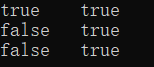我们看下它的反编译代码

Integer integer = Integer.valueOf(10);
Integer integer1 = Integer.valueOf(10);
Integer integer2 = new Integer(20);
Integer integer3 = new Integer(20);
Integer integer4 = Integer.valueOf(128);
Integer integer5 = Integer.valueOf(128);
System.out.println((new StringBuilder()).append(integer == integer1).append("\t").append(integer.equals(integer1)).toString());
System.out.println((new StringBuilder()).append(integer2 == integer3).append("\t").append(integer2.equals(integer3)).toString());
System.out.println((new StringBuilder()).append(integer4 == integer5).append("\t").append(integer4.equals(integer5)).toString());

首先，我们查看Integer的valueOf()方法的源码

    public static Integer valueOf(int i) {
if (i >= IntegerCache.low && i <= IntegerCache.high)
return IntegerCache.cache[i + (-IntegerCache.low)];
return new Integer(i);
}

再查看下Integer的内部类IntegerCache的cache数组成员、low、high成员

        static final int low = -128;
static final int high;
static final Integer cache[];

static {
// high value may be configured by property
int h = 127;
String integerCacheHighPropValue =
sun.misc.VM.getSavedProperty("java.lang.Integer.IntegerCache.high");
if (integerCacheHighPropValue != null) {
try {
int i = parseInt(integerCacheHighPropValue);
i = Math.max(i, 127);
// Maximum array size is Integer.MAX_VALUE
h = Math.min(i, Integer.MAX_VALUE - (-low) -1);
} catch( NumberFormatException nfe) {
// If the property cannot be parsed into an int, ignore it.
}
}
high = h;

cache = new Integer[(high - low) + 1];
int j = low;
for(int k = 0; k < cache.length; k++)
cache[k] = new Integer(j++);

// range [-128, 127] must be interned (JLS7 5.1.7)
assert IntegerCache.high >= 127;
}

可以发现，只要Integer类第一次被使用到，Integer的静态内部类就被加载，加载的时候会创建-128到127的Integer对象，同时创建一个数组cache来缓存这些对象。当使用valueOf()方法创建对象时，就直接返回已经缓存的对象，也就是说不会再新建对象；当使用new关键字or使用valueOf()方法创建小于-128大于127的值对象时，就会创建新对象。

//2、包装类中的缓存机制
Integer num3 = 10;
Integer num4 = 10;
Integer num5 = new Integer(20);
Integer num6 = new Integer(20);
Integer num7 = 128;
Integer num8 = 128;


由于num3、num4都小于等于127，它们指向的是同一个缓存的Integer对象，所以用==进行比较的结果是true；num5、num6由于使用new关键字指向的是两个不同的新对象，结果为false；num7、num8虽然是采用自动装箱的方式，但执行valueOf()方法的时候，由于不满足条件i >= IntegerCache.low && i <= IntegerCache.high，而同样新建了两个不同的新对象，结果同样是false。

接着，我们再来看看源码中Integer的equals()方法的实现

    public boolean equals(Object obj) {
if (obj instanceof Integer) {
return value == ((Integer)obj).intValue();
}
return false;
}

可见equals()方法比较的是Integer对象的值，而不是像==一样比较的是对象是否是同一个对象。所以，当需要比较两个Integer对象的值是否相等时，记住要用equals()方法。用==比较的话由于缓存机制的存在，可能产生一些让人困扰的结果。

此外，在8种包装类型中，有缓存区的有Character、Byte、Short、Integer、Long，而且它们的实现方式基本一样，都是-128到127的缓存范围。Boolean虽然没有缓存区，但是因为只有两个值true、false，所以Boolean在成员变量中就创建了两个相应的对象。没有缓存区的只有Float、Double，之所以没有原因很简单，即便是0到1这么小的范围，浮点数也有无数个，使用缓存区缓存它们不具备可能性和实用性。

缓存区的存在使得常用的包装类对象可以得到复用，这有利于提升性能。当我们需要创建新对象的时候再new一个，增加了灵活性。

### 四、包装类的四则运算、位运算、比较运算、逻辑运算

1、四则运算和位运算

//四则运算、位运算
Integer num9 = 1;
Integer num10 = 2;
Integer num11 = num9 + num10;
Short num12 = 5;
Integer num13 = num9 + num12;
Long num14 = num9 + 10L;
System.out.println(num9 << 1);	//位运算
System.out.println(num9 +"	"+ num10 +"	"+ num11 +"	"+ num12 +"	"+ num13 +"	"+ num14);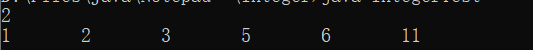反编译结果如下

        Integer integer = Integer.valueOf(1);
Integer integer1 = Integer.valueOf(2);
Integer integer2 = Integer.valueOf(integer.intValue() + integer1.intValue());
Short short1 = Short.valueOf((short)5);
Integer integer3 = Integer.valueOf(integer.intValue() + short1.shortValue());
Long long1 = Long.valueOf((long)integer.intValue() + 10L);
System.out.println(integer.intValue() << 1);
System.out.println((new StringBuilder()).append(integer).append("\t").append(integer1).append("\t").append(integer2).append("\t").append(short1).append("\t").append(integer3).append("\t").append(long1).toString());


可以看到Integer num11 = num9 + num10; 这一句被划分为3个步骤：将两个Integer对象分别进行拆箱；将拆箱得到的两个int数值相加求其和；将和值进行装箱，从而将num11指向缓存数组中值为3的Integer对象。

而Short num12 = 5; 这一句则先将5强制转换成short类型，再将其装箱把值为5的Short对象的引用赋给num12。

而Integer num13 = num9 + num12; 这一句除了Integer num11 = num9 + num10;的3个步骤，中间还有short+int=int的类型自动提升的过程。

而Long num14 = num9 + 10L; 这一句Integer num11 = num9 + num10;的3个步骤，中间还有强制类型转换的过程。需要注意的是，如果是Long num14 = num9 + num10; 的话就会出现类型不匹配的错误，因为num9、num10拆箱之后相加的和是int类型，而Long.valueOf(long)需要的形参是long类型，自然会出错。我们也可以看到，当包装类型对象和基本类型数据进行四则运算的时候，对象是会被拆箱的，然后再按基本类型数据的运算规则进行运算。

另外，如果仅仅是打印两个包装类型对象求和的结果，是不会有将和值重新转换成该包装类型的步骤的，如下面所示

System.out.println(num9 + num10);
System.out.println(integer.intValue() + integer1.intValue());

这里还需要注意一点，尽管基本类型与自动类型提升/强制类型转换，包装类是没有类似的用法的。下面的做法是错的。

Short num3 = 10;
Integer num4 = num3;	//错误: 不兼容的类型: Short无法转换为Integer
Long num5 = (Long)num4;	//错误: 不兼容的类型: Integer无法转换为Long
Double num6 = num5;	//错误: 不兼容的类型: Long无法转换为Double

小结：不同包装类型对象是不能直接转换的，不过有两种途径可以代替：一种是上面讨论的不同包装类对象进行四则运算后赋给某一种类型；另一种就是利用包装类的方法

Integer a = 20;
Long b = a.longValue();
Short c = b.shortValue();
System.out.println(a +"	"+ b +"	"+ c);

2、比较运算和逻辑运算

Integer num9 = 100;
Integer num10 = 200;
Short num11 = 50;
Long num12 = 50L;
System.out.println((num9<num10) +"	"+ (num9<200) +"	"+ (num9<num11) +"	"+ (num9<num12) +"	"+ (num9<10L));

反编译结果为

Integer integer = Integer.valueOf(100);
Integer integer1 = Integer.valueOf(200);
Short short1 = Short.valueOf((short)50);
Long long1 = Long.valueOf(50L);
System.out.println((new StringBuilder()).append(integer.intValue() < integer1.intValue()).append("\t").append(integer.intValue() < 200).append("\t").append(integer.intValue() < short1.shortValue()).append("\t").append((long)integer.intValue() < long1.longValue()).append("\t").append((long)integer.intValue() < 10L).toString());


可以看到，两个同类型的包装类对象进行比较时比较的其实是各自的基本类型数值，如num9 < num10；两个不同类型的包装类对象进行比较时则在比较基本类型数值之前，会有类型提升or强制类型转换，如num9 < num11，num9 < num12。

当想比较两个对象是否相等时，注意要使用equals()方法，从前面的讨论也知道，使用==的话比较的其实是引用的对象是否同一个，一般不满足我们的需求。

Integer num13 = new Integer(100);
System.out.println(num9.equals(num13) +"	"+ num9.equals(50));

反编译结果为

Integer integer2 = new Integer(100);
System.out.println((new StringBuilder()).append(integer.equals(integer2)).append("\t").append(integer.equals(Integer.valueOf(50))).toString());


逻辑运算举例：

System.out.println((num9&1));

反编译结果为

System.out.println(integer.intValue() & 1);

### 五、包装类作为方法的形参、返回值

//包装类作为方法的形参、返回值
public static Integer intToInteger(int i) {
return i;
}
public static int integerToInt(Integer i) {
return i;
}

反编译结果为

    public static Integer intToInteger(int i)
{
return Integer.valueOf(i);
}

public static int integerToInt(Integer integer)
{
return integer.intValue();
}

### 六、包装类作为集合的元素

//包装类作为集合元素
List list = new ArrayList();
Iterator it = list.iterator();
while (it.hasNext()) {
System.out.println(it.next());
}

反编译结果为

ArrayList arraylist = new ArrayList();
for(Iterator iterator = arraylist.iterator(); iterator.hasNext(); System.out.println(iterator.next()));

                List<Integer> list = new ArrayList<>();
for (int i=0; i<5; i++) {
}
Iterator it = list.iterator();
while (it.hasNext()) {
System.out.println(it.next());
}

反编译结果为

        ArrayList arraylist = new ArrayList();
for(int i = 0; i < 5; i++)

for(Iterator iterator = arraylist.iterator(); iterator.hasNext(); System.out.println(iterator.next()));

### 七、包装类使用过程中有可能引起的空指针异常

//注意包装类可能产生的空引用异常
Boolean flag1 = false;
System.out.println(flag1?"命题为真":"命题为假");
Boolean flag2 = null;
System.out.println(flag2?"命题为真":"命题为假");
Boolean flag3 = true;

运行结果为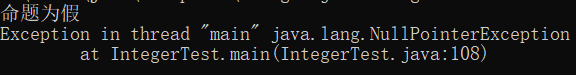这里只是简单演示空指针异常。平时使用时需要注意这一点，比如当Boolean的对象作为形参时，在方法执行体的头部需要做下null检测。

上述代码的反编译结果为

        Boolean boolean1 = Boolean.valueOf(false);
System.out.println(boolean1.booleanValue() ? "\u547D\u9898\u4E3A\u771F" : "\u547D\u9898\u4E3A\u5047");
Boolean boolean2 = null;
System.out.println(boolean2.booleanValue() ? "\u547D\u9898\u4E3A\u771F" : "\u547D\u9898\u4E3A\u5047");
Boolean boolean3 = Boolean.valueOf(true);

可见三目运算符的条件表达式的位置一定是boolean值，如果你传入的是Boolean对象，则会自动拆箱转换为boolean值。

另外，三目运算符的其他两个表达式位置也是如此，会把包装类对象转换为相应的基本类型对象。

### 八、为什么需要包装类？有了包装类又为什么要保留基本数据类型？（包装类的优缺点）

为什么需要包装类？

首先，Java语言是一个面向对象的语言，但是Java中的基本数据类型却是不面向对象的，将每个基本数据类型设计一个对应的类进行代表，这种方式增强了Java面向对象的性质。

其次，如果仅仅有基本数据类型，那么在实际使用时将存在很多的不便，很多地方都需要使用对象而不是基本数据类型。比如，在集合类中，我们是无法将int 、double等类型放进去的，因为集合的容器要求元素是Object类型。而包装类型的存在使得向集合中传入数值成为可能，包装类的存在弥补了基本数据类型的不足。

此外，包装类还为基本类型添加了属性和方法，丰富了基本类型的操作。如当我们想知道int取值范围的最小值，我们需要通过运算，如下面所示，但是有了包装类，我们可以直接使用Integer.MAX_VALUE即可。

//求int的最大值
int max = 0;
int flag = 1;
for (int i=0; i<31; i++) {
max += flag;
flag = flag << 1;
}
System.out.println(max +"	"+ Integer.MAX_VALUE); //2147483647      2147483647

为什么要保留基本数据类型？

我们都知道在Java语言中，用new关键字创建的对象是存储在堆里的，我们通过栈中的引用来使用这些对象，所以，对象本身来说是比较消耗资源的。对于经常用到的类型，如int等，如果我们每次使用这种变量的时候都需要new一个对象的话，就会比较笨重了。所以，Java提供了基本数据类型，这种数据的变量不需要使用new在堆上创建，而是直接在栈内存中存储，因此会更加高效。

展开全文自动装箱
• ## Java包装类

千次阅读 2021-03-08 05:57:02
1、Java 包装类Java有8基本数据类型：整型(byte、short、int、long)、浮点型(float、double)、布尔型boolean、字符型char，相对应地，Java提供了8种包装类Byte、Short、Integer、Long、Float、Double、Boolean、...
• Java基本类型包装类：基本数据类型 基本类型包装类byte Byteshort Shortint Integerlong Longfloat Floatdouble Doublechar Charac...
• 二、八种基本数据类型 三、8种基本类型及其包装类 Java为这8种基本数据类型，提供了对应的包装类。每个包装类都对相应的基础类型有操作方法，也有几种类型的转换。大家可以对应api文档去学习，这里就不详细说明了。...
• 今天就为大家介绍一下java包装类是什么意思，以及它包括哪些内容。首先，由于java中的数据类型int，double等不是对象，所以无法通过向上转型得到Object提供的方法，而像String却可以，原因是String是一个对象而...
• 一、java八种基本数据类型对应的包装类型：   原始类型 包装类 原始类型所占的字节数  short Short 2个字节  int Integer 4个字节  long Long 8个字节  float Float 4个字节  double Do8大基本数据类型
• Java大基本类型及包装类，分别是： byte 包装类是 Byte short包装类是 Short int 包装类是 Integer long 包装类是 Long float 包装类是 Float double 包装类是 Double char 包装类是 Character boolean...
• 包装类概述 二 . 装箱与拆箱 一 . 包装类概述 我们先来看两个问题 1.什么是基本类型包装类? 这些类封装了一个相应的基本数据类型数值，并为其提供了一系列操作方法。例如Integer类包装一个int值 2.我们都...开发语言 后端
• ## Java包装类型有几种

千次阅读 2021-03-14 16:35:05
【相关学习推荐：java基础教程】Java包装类型有8，分别是：Java中的基本类型功能简单，不具备对象的特性，为了使基本类型具备对象的特性，所以出现了包装类，就可以像操作对象一样操作基本类型数据。一、基本类型...
• 八种基本数据类型以及包装类1. 基本数据类型 byte 占用1个字节（8位），范围：-2^7~2^7-1 short 占用2个字节（16位)，范围：-2^15~2^15-1 int 占用4个字节（32位），范围：-2^31~2^31-1 long 占用8个字节（64位）...
• Double 在对象中包装了一个基本类型 double 的值。Double 对象包含一个 double 类型的字段。此外，该还提供了多个方法，可以将 double 类型与 String 类型相互转换，同时 还提供了处理 double 类型时比较常用...
• Float 在对象中包装了一个基本类型 float 的值。Float 对象包含一个 float 类型的字段。此外，该提供了多个方法，能在 float 类型与 String 类型之间互相转换，同时还提供了处理 float 类型时比较常用的常量和...
• Java是面向对象的语言，但并不是“纯面向对象”的，因为我们经常用到的基本数据类型就不是对象。但是我们在实际应用中经常需要将基本数据转化成对象，以便于操作。比如：将基本数据类型存储到Object[]数组或集合中的...
• ## Java数据类型—包装类

万次阅读 多人点赞 2020-12-16 16:16:27
1. 为什么需要包装类JAVA是面向对象的语言，很多类和方法中的参数都需使用对象(例如集合)，但基本数据类型却不是面向对象的，这就造成了很多不便 2. 拆装箱的概念：将基本数据类型转为包装类的过程叫装箱，将包装...jvm
• ## Java包装类是什么

万次阅读 多人点赞 2018-04-26 14:29:49
包装类就是将基本的数据类型以及一些辅助方法封装到类中，例如 class IntDemo { private int num; public IntDemo(int num) { this.num = num; } public int intValue() { return this.num; } } Java...拆箱与装箱
• 1 正则表达式Regex 1.1 概述 正确的字符串格式规则。 常用来判断用户输入的内容是否符合格式的要求，注意是严格区分大小写的。 1.2 常见语法 ...创建: TestRegex.java package cn.tedu.api; impo正则表达式 自动装箱
• ## JAVA中包装类的作用

万次阅读 多人点赞 2019-04-16 12:04:37
为了使用方便和解决这个不足，在设计类时为每个基本数据类型设计了一个对应的类进行代表，这样八种基本数据类型对应的类统称为包装类(Wrapper Class)，包装类均位于java.lang包。 二、包装类的用途 为了使用方便Java...
• Java初学阶段，想必很多人都会先学Java中的一些基本类型以及他们的包装类,如下 int --> Integer long --> Long double --> Double ...等等 很多人在学的时候感觉这用处不大啊，也不知道什么时候用，该...
• 为了解决这个不足，Java在设计类时为每个基本数据类型设计了一个对应的类进行代表，这样个和基本数据类型对应的类统称为包装类(Wrapper Class)。 基本类型对应的包装类如下： 在这个类名中，除了Integer和...
• Java有8基本数据类型，为什么又要出现对应的8种包装类：1、Java的8基本数据类型不支持面向对象编程机制2、8基本数据类型不具备“对象”的特性：没有成员变量、方法可供调用3、例如：某个方法需要Object类型的...
• 判断字符串 ...其他包装类，用法一致 Byte 、Short、Integer、Long、Float、Double、Character、Boolean、String 判断数组和集合 1 数组（精确） dataArray instanceof String[] dataArray instanc...
• 一、java八种基本数据类型对应的包装类型： 原始类型 包装类 原始类型所占的字节数 short Short 2个字节 int Integer 4个字节 long Long 8个字节 float Float 4个字节 double Double ...
• java八大基本类型： 整型： byte： byte数据类型是8位、有符号的，以二进制补码表示的整数； 最小值是-128（-2^7）； 最大值是127（2^7-1）； 默认值是0； byte类型用在大型数组中节约空间，主要代替整数，...
• 最近在业务中碰到了 Integer 默认值的判空 导致的业务逻辑问题，引起了兴趣，针对基本数据类型和其包装类有什么区别？测试过程中应该注意哪些问题呢？...回答这些问题前，我们先来看看java中基本数据类型和包装类有哪些测试 基本数据类型
• Byte类/包装类包装类是一个常量类，可实例化（意义：帮助对应的数据类型进行数据类型）java数据类型包括内置数据类型和引用数据类型内置数据类型：含6中数字类型（四个整数型，两个浮点型），一字符类型，还有布尔...
• Java提供了两个类型系统，基本类型与引用类型，使用基本类型在于效率，然而很多情况，会创建对象使用，因为对象可以做更多的功能，如果想要我们的基本类型像对象一样操作，就可以使用基本类型对应的包装类，如下： ...
• ## java包装类 Boolean

千次阅读 2018-07-28 21:03:47
Boolean 一个Boolean类型的对象只包含一个类型为boolean的字段，此类还为boolean和String的相互转换提供了很多方法。 构造方法 Boolean(boolean value); 该方法创建一个表示value参数的Boolean对象 ......

# java八种包装类java 订阅